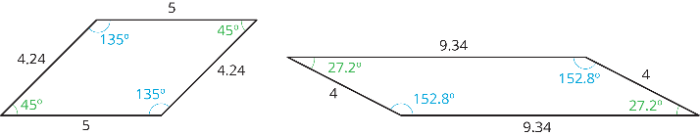# Parallelograms: IM Lesson 6.1.4

This GeoGebra activity has been created from this lesson authored by Illustrative Mathematics.

## Study the figures above.

How many sides does a parallelogram have?

Do you notice anything about opposite angles of a parallelogram? If so, describe.

Do you notice anything about opposite sides of a parallelogram? If so, describe.

## An empty parallelogram is shown on the left. Use any 1 or more of the colored polygons to help find its area.

What is the area of the parallelogram above? Explain your reasoning.

## Change the parallelogram by dragging the green points at its vertices. Then use any 1 or more of the colored polygons to help find its area.

﻿If you used the polygons on the side, how were they helpful? If you did not, try using one or more of the polygons to show another way to find the area of the parallelogram in the app above.

## Use the parallelograms shown in A and B to help answer the questions below.

What is the area of parallelogram A? Explain your thought process.

What is the area of parallelogram B? Explain your reasoning.

## For the question below, refer to parallelogram C shown here.

What is the area of parallelogram C? Explain how you know.

## SUMMARYA parallelogram is a quadrilateral (it has four sides). The opposite sides of a parallelogram are parallel. It is also true that the opposite sides of a parallelogram have equal length, and the opposite angles of a parallelogram have equal measure.
There are several strategies for finding the area of a parallelogram: